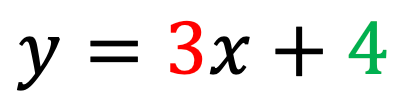// StartMathJax Script window.MathJax = {loader: {load: [ 'input/asciimath', 'ui/lazy', 'output/chtml', 'ui/menu']} }; (function() { var script = document.createElement('script'); script.src = "https://cdn.jsdelivr.net/npm/mathjax@3/es5/startup.js"; script.async = true; document.head.appendChild(script); })(); ---------- (Different files) ---------- // UpdateTypeset Script config = { attributes: true, childList: true, subtree: true }; // Callback function to execute when mutations are observed callback = (mutationList, observer) => { for (mutation of mutationList) { if (mutation.type === 'childList') { console.log('A child node has been added or removed.'); MathJax.typeset(); } else if (mutation.type === 'attributes') { console.log(The \${mutation.attributeName} attribute was modified.); } } }; // Create an observer instance linked to the callback function observer = new MutationObserver(callback); document.onreadystatechange = () => { if (document.readyState === 'complete') { console.log("Loaded fully according to readyState") targetNode = document.getElementById('content-wrapper') console.log(targetNode) // Start observing the target node for configured mutations observer.observe(targetNode, config); } }
top of page
Search

# A Couple of Points on Straight Line Graphs...

Here we look at a great way to construct/sketch a straight line graph that requires just two points.

## The two-point gradient and y-intercept method

The equation for a straight line isWhere x and y are variables that relate to the x and y axes of a graph.

The gradient (slope) of the line is given by m - this is the number immediately before the x (it's known as the coefficient). If the coefficient is a negative value, the gradient will slope downwards on a graph.

The c term is the y-intercept - the point on the y-axis where the line will cross.

Look at the following equation...If it can be manipulated so that it has the form y = mx + c, it can be graphed.In this case, the line will have a gradient of 3 and will cross the y-axis at y = 4.

The line can be graphed quickly by plotting just two points.

The easiest point to plot is the y-intercept. This occurs when x = 0 so the coordinates will be (0, 4)The y-intercept

Another point on the graph is needed and it can be found using the value for the gradient, 3.

The gradient is usually found by calculating......the change in y divided by the change in x for 2 points on a line.

Looking at the equation, this is already done. The value 3 can be represented as .This just means that for every unit increase in a value for x, there will be 3-fold unit increase in the value of y.

Starting with the y-intercept coordinate, move 1 place along the x-axis and 3 places up the y-axis, to get a second coordinate as follows:

The x value will move from 0 to 1 and the y value will move from 4 to 7 (increasing by 3). The new coordinate will be (1, 7).Graph showing two coordinates

Complete by lining up a straight edge to the two coordinates and pencil in the line. This line represents the equation and can be read off to find the corresponding values for the variables x and y.Graph of y = 3x + 4

## The x-intercept

The point where the line crosses the x-axis can also be found from the equation...Set the y value to 0 (the value for y when the value for x lies directly on the x-axis)...Make x the subject...## Summary

• Find and plot the y-intercept from the equation for a straight line

• Use the coefficient of x to find a second point on the graph

• Line up the points and draw a straight line!

Have a go at plotting the following. You can construct your own graphs or use the Graphing Blank provided below.Graph paper

2x Graphing Blank
.pdf

Here are the solutions

Solutions to Straight-line Graph Problems
.pdf